The Golden Ratio Triangles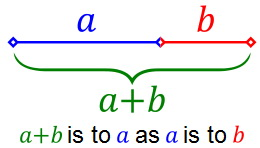Figure 1 The golden ratio definition Since the Sri Yantra is made of triangles it is worth while spending some time learning a little more about triangles, more specifically triangles in the context of the famous golden ratio. We have to start with the golden ratio which is also known as the phi ratio or the golden proportion. It is the only ratio that satisfies the definition a+b is to a as a is to b show in figure 1. In simpler terms this means that a+b / a = a /b. If we fold b over a it will divide it in the golden ratio. If we take the smaller part and fold it again it will divide the line in the same proportion over and over again. It's a perfect self-embedding proportion which explains why most of our limbs are divided in the proportion of the golden ratio.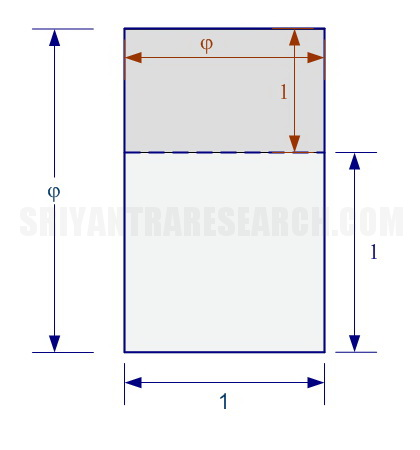figure 2. The golden rectangle If this seems too abstract lets look at it in 2 dimensions. Figure 2 shows a simple golden rectangle with sides 1 x φ. If we divide this rectangle again by drawing a line of length equal to the shorter side (length 1) we end up with a smaller golden ratio rectangle (dark gray). We can repeat this operation with the smaller rectangle and end up once again with a smaller golden rectangle and a square. This shows the fractal (self similiarity) and self embeddedness properties of the godlen ratio.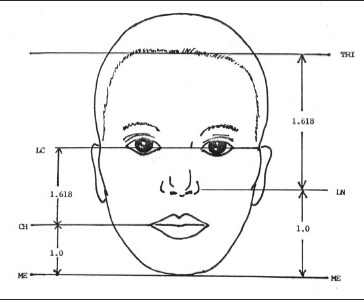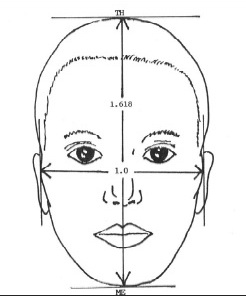Figure 3 Golden ratio beauty mask Our perception of beauty is actually defined by the the golden ratio. An attractive person is attractive because their proportions are closer to the golden ratio. In nature the Fibonacci numbers are a close approximation of the golden ratio since fractions are not always possible in the real world. The Golden proportion is 1.6180.. also noted by the greek letter phi (φ). The inverse of phi (1/φ) is noted with capital phi (Φ) and is equal to 0.6180... That's one of the many surprising property of the golden ratio φ - 1 = 1 / φ. In the case of the golden rectangle things are simple. You draw a rectangle with side 1 and 1.6180... You will find many methods on the internet (wikipidia) that will show you how to draw it precisely using a straightedge and compass. But since the Sri Yantra is made of 9 interlocking triangles we are mainly interested in triangles. There is only one way to draw a golden ratio rectangle. A rectangle has only two degrees of freedom: width and height. But with a triangle there is width, height, and length of the slanted side. Three degrees of freedom. We will also see that other variations are also possible when we use a right angle triangle versus an isosceles one. This gives rise to many variations of "golden ratio" triangles. In this article will will look at seven different ones and their characteristics. The King and Queen Lets start with the King and Queen of the golden ratio triangles. These two are the real thing. These two triangles are what is usually implied by "golden ratio triangle".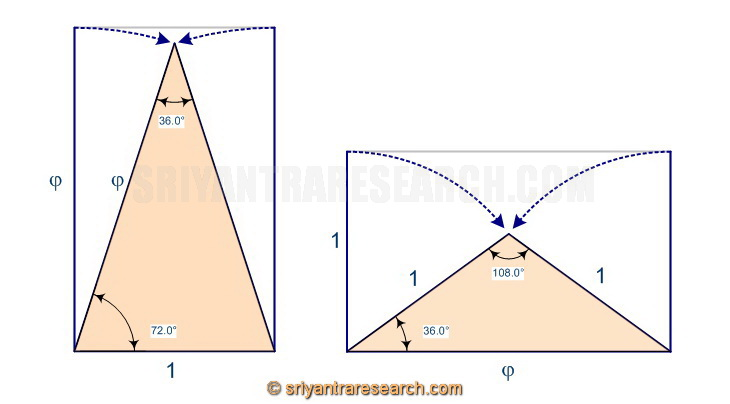Figure 4 Creating the golden triangles from the golden rectangle These two golden triangles can be created easily from the golden rectangle by removing one of the sides and collapsing the two open sides until they meet in the middle as shown in figure 4. Interestingly the angles of these two triangles are all multiples of 36.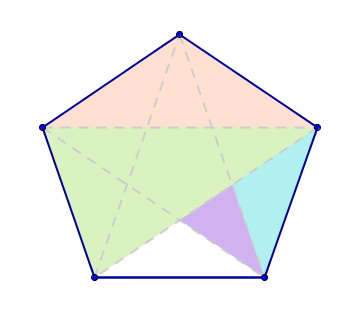Figure 5 Golden triangles in the pentagon Another way of generating golden triangles is to draw a pentagon. The pentagon more than any other figure is associated with the golden ratio. When joining the five apex of a pentagon we end up with a multitude of golden ratio triangles. The colored areas in figure 5 show the two golden triangles showing up in two different sizes in the pentagon.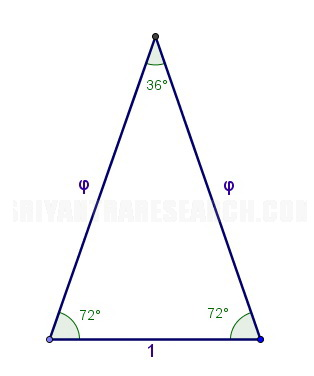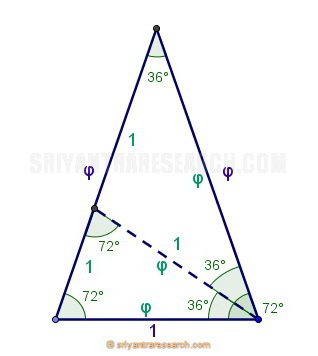Figure 6 Golden triangle King Lets look at those two triangles in more detail and see what makes them so special. In the image above we see that this triangle has two angles of 72 degrees and one angle of 36 degrees. The angles are all multiples of 36. If the base is of length 1 then the slanted sides will be of length 1.6180.. which is symbolized by the greek letter phi (φ). This is why it is also called the phi ratio. If we run a line that divides one of the 72 degrees angle in two (bisecting of the angle) we see that another smaller golden ratio triangle appears. This shows the self similar property of the golden ratio at work. The same self replication effect is noticed with the golden rectangle.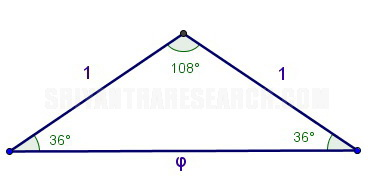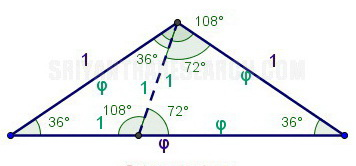Figure 7 Golden triangle Queen Another golden ratio triangle can obviously be created by making the two slanted sides length 1 and the base length 1.6180. This produces a flatter triangle with the same angles 36 and 72 degrees. Here again we can subdivide this triangle (shown with the dotted line) this time by dividing the 72 degree angle by 1/3. We end up with a smaller triangle with the same proportions on the left side. On the right side we have a triangle similar to the first one we created. We can keep subdividing like this forever which will create a golden ratio spiral. Another pattern widely used in nature. The Golden Cousins The two triangles we have studied in the previous section are the real golden ratio triangles. However it is possible to create a variation by making a triangle with a base of unit length and a height of 1.6180 (instead of the side length). It is very similar to the first triangle we looked at but it doesn't have the self replicating and fractal qualities which is typical of the real golden ratio triangles.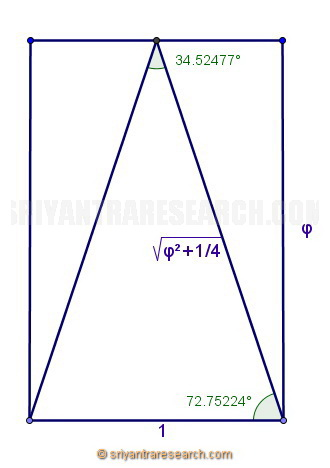Figure 8 So far we have been using isosceles triangles. Symmetrical triangles along the vertical axis. We can also use right angle triangles which gives rise to two more golden ratio cousins.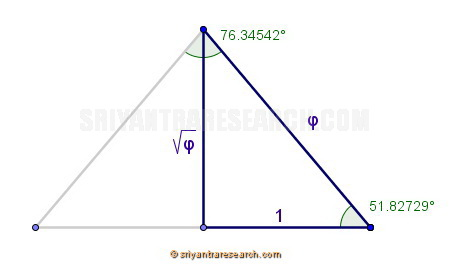Figure 9 Golden triangle used for the pyramid of Giza Now since we are using a right angle triangle we have three side of different lengths instead of two as in an isosceles triangle. This gives rise to more ways to introduce the golden ratio in a triangle. The first can be seen in the image above. In this golden ratio triangle we use the base as unit length and the slanted side as phi length. This interestingly results in a height of square root of phi. One more sign of the magic of the golden ratio. If we create a mirror image of this triangle we end up with an isosceles triangle of base 2, a height of square root of phi and sides of length phi. This by the way is really close to the shape of the famous great pyramid of giza.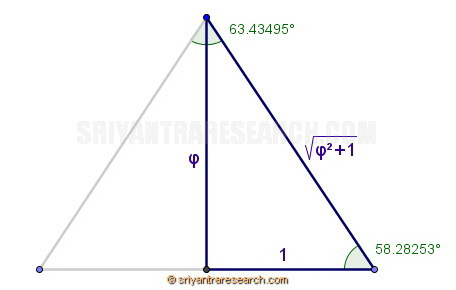Figure 10 The second variation can be achieved by giving the triangle a height of phi and base of one. This results in a triangle which is slightly higher than the previous one since the angle is of 58.3 degrees instead of 51.8 degrees. It is now easy to see why the first one was selected as the shape of the pyramid in giza. It makes for a pyramid that is not too high or too low, stable and pleasant to look at. The Distant Cousins Two other sub variations are also possible. These would be consider the most inferior type since none of their sides measure exactly phi.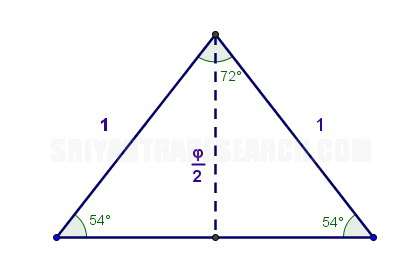Figure 11 Golden triangle is a subset of the Queen triangle. This variation uses a height of phi/2 and a side of length 1. We see that this triangle is actually a subset of the second one we look at. You can see that we have the same angles of 36 and 108 degrees. It is half of that triangle. We see that in this triangle we have angles that are multiples of 18 as with the first two triangles.Figure 12 This last triangle completes the series of multiples of 18. We see that the phi ratio and it's conjugate (capital phi) shows up in all three lengths. We now have triangles with a smaller angle of 18, 36, 54, 72 degrees which are all multiples of 18. The Family Reunion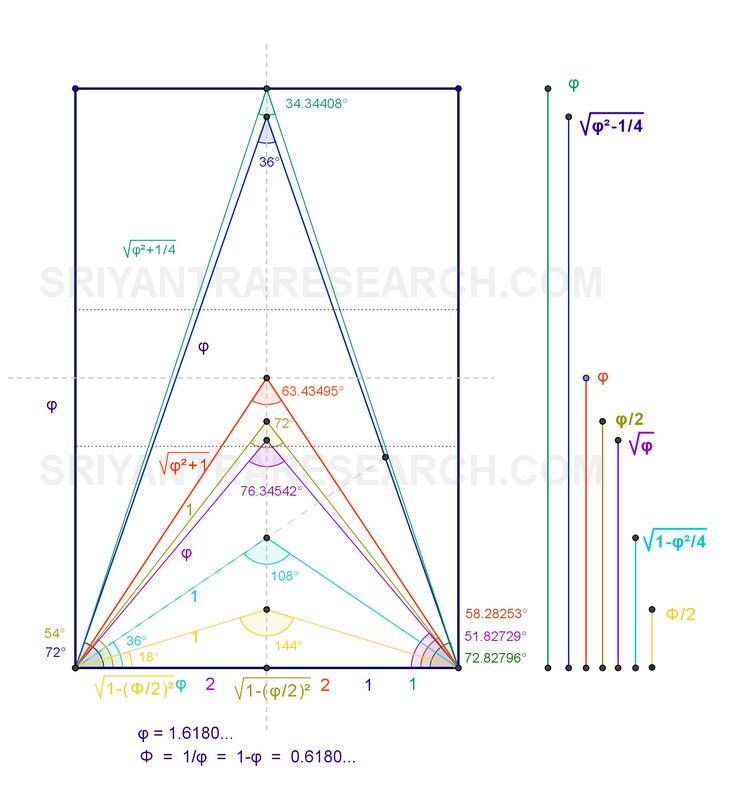Figure 13 The golden triangles inside a golden rectangle. This figure shows all the golden ratio triangles fitted inside a golden rectangle. We could divide the golden ratio in three families. First we have the triangles with even angles that are a multiple of 18. This can be further divided in two groups. The King and Queen of the golden ratio triangles are the first two that we generated by collapsing the sides of the golden rectangle. The sides of these two triangles have length 1 or φ. A third group would include the triangles that have irrational angles: 51.82..., 58.28..., 72.82... The Equilateral TriangleFigure 14 The Equilateral triangle We should also mention the equilateral triangle as a major player in the triangle arena. It doesn't have any golden proportions but it is a basic component of nature because of it's perfect symmetry and simplicity. We notice that it shows up in many of the platonic solids that are the building block of three dimensional geometry. All crystal shapes for example can be derived from the platonic solids.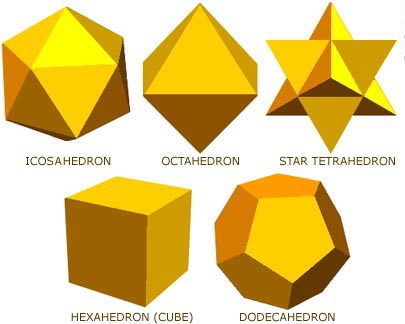Figure 15 The platonic solids As we can see in this figure it is a building block of 3 of the 5 platonic solids. The equilateral triangle is the simplest geometric surface because it has the minimum number of sides. Any image or shape can be expressed as a group of triangles (triangulation). It is also interesting to note how the number 3 is considered holy in many religions. The holy trinity comes to mind as well as the many trinities of the vedic knowledge (Vishnu, Shiva, Brahman), (Rishi, Devata, Chanda), (Sattva, Rajas, Tamas), (Vata, Pitta, Kapha), etc.